Difference between revisions of "User:Ddcampayo/Sandbox"

Hi world. --Dduque 14:18, 23 April 2008 (CEST)

 f66 This user uses fortran 66
 C++ This user uses C++

<DPL>

category=Models
ordermethod=counter
order=descending
count=5
allowcachedresults=true

</DPL>

Notation used:

•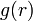$g(r)$ is the pair distribution function.
•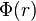$\Phi(r)$ is the pair potential acting between pairs.
•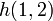$h(1,2)$ is the total correlation function.
•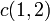$c(1,2)$ is the direct correlation function.
•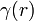$\gamma (r)$ is the indirect (or series or chain) correlation function.
•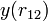$y(r_{12})$ is the cavity correlation function.
•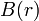$B(r)$ is the bridge function.
•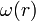$\omega(r)$ is the thermal potential.
•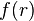$f(r)$ is the Mayer f-function.